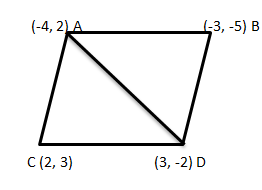Courses
Courses for Kids
Free study material
Offline Centres
MoreLast updated date: 03rd Dec 2023
Total views: 381.9k
Views today: 3.81k

# Find the area of the quadrilateral whose vertices taken in order, are $\left( { - 4, - 2} \right),\left( { - 3, - 5} \right),\left( { - 3, - 2} \right){\text{ and }}\left( {2,3} \right)$.Verified
381.9k+ views
Hint- To solve this question we will use the formula given by $\Delta = \dfrac{1}{2}\left| {{y_1}({x_2} - {x_3}) + {y_2}({x_3} - {x_1}) + {y_3}({x_1} - {x_2})} \right|$ , we will divide the quadrilateral into two triangles and then apply the formula.

Complete step-by-step solution -
Given that vertices of quadrilateral are $\left( { - 4, - 2} \right),\left( { - 3, - 5} \right),\left( { - 3, - 2} \right){\text{ and }}\left( {2,3} \right)$Let $A\left( { - 4, - 2} \right),B\left( { - 3, - 5} \right),C\left( { - 3, - 2} \right){\text{ and D}}\left( {2,3} \right)$ be the vertices of the quadrilateral.
As we know that the area of the quadrilateral ABCD= area of $\Delta ABC$ + area of $\Delta ACD$
Area of $\Delta ABC$ is given by
$= \dfrac{1}{2}\left| {{y_1}({x_2} - {x_3}) + {y_2}({x_3} - {x_1}) + {y_3}({x_1} - {x_2})} \right|$
In $\Delta ABC$
${x_1} = - 4,{x_2} = - 3,{x_3} = 3,{y_1} = - 2,{y_2} = - 5,{y_3} = - 2$
Substituting above values in the formula, we get
$= \dfrac{1}{2}\left| { - 2( - 3 - 3) - 5(3 + 4) - 2( - 4 + 3)} \right| \\ = \dfrac{1}{2}\left| { - 2( - 6) - 5(7) - 2( - 1)} \right| \\ = \dfrac{1}{2}\left| {12 - 35 + 2} \right| \\ = \dfrac{1}{2}\left| { - 21} \right| \\ = 10.5{\text{ sq}}{\text{.units}} \\$

Area of $\Delta ACD$ is given by
$= \dfrac{1}{2}\left| {{y_1}({x_2} - {x_3}) + {y_2}({x_3} - {x_1}) + {y_3}({x_1} - {x_2})} \right|$
In $\Delta ACD$
${x_1} = - 4,{x_2} = 3,{x_3} = 2,{y_1} = - 2,{y_2} = - 2,{y_3} = 3$
Substituting above values in the formula, we get
$= \dfrac{1}{2}\left| { - 2( - 3 - 2) - 2(2 + 4) - 3( - 4 - 3)} \right| \\ = \dfrac{1}{2}\left| { - 2 - 12 - 21} \right| \\ = \dfrac{1}{2}\left| { - 35} \right| \\ = 17.5{\text{ sq}}{\text{.units}} \\$
Therefore, the area of the quadrilateral is = $10.5 + 17.5 = 28{\text{ sq}}{\text{. units}}$
Hence, the area of the quadrilateral is $28.0{\text{ sq}}{\text{. units}}$

Note- The given problem is the coordinate geometry problem and the vertices of the quadrilateral are given. In order to solve such questions, try to break the quadrilateral in two triangles and apply the formula to calculate the area of the triangle.